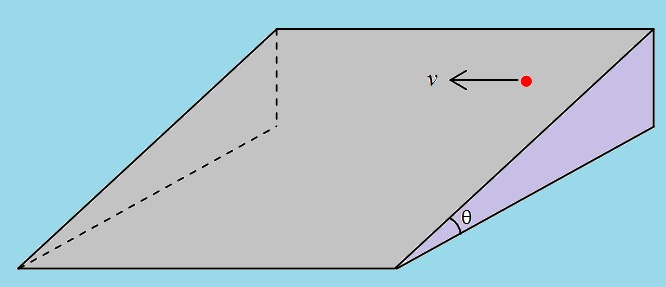# Inclined Terminal.A particle is placed on a fixed rough inclined plane which makes an angle $\theta$ from ground. The co-efficient of friction between between the particle and the inclined plane is $\mu = tan\theta$.

The particle is given a velocity $v$ along the line which is common to the inclined plane and the horizontal plane at that height.

Find the magnitude of terminal velocity (in $ms^{-1}$) attained by the particle.

Given Data : $v = 9 ms^{-1}$. Assume that the inclined plane is very large so that the particle never leave that plane.

Try more from my set Classical Mechanics Problems.

×